Chapter 3.3 Solution of the LS Problem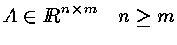( Ax = b is called the over-determinate system ) Find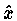s.t.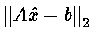is minimal .

( min is the sense that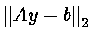for all y )

[Thm] If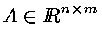,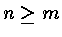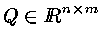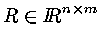s.t. A=QR and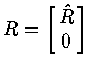<Proof>Let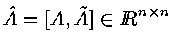is  choosen arbitrary .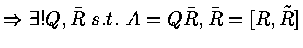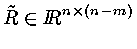( upper triangle )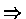A=QR

R  has the formExample :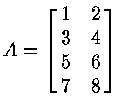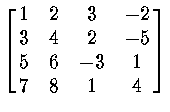=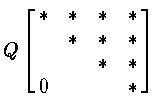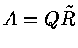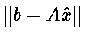( Q  is  orthonogal  )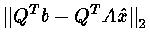=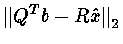==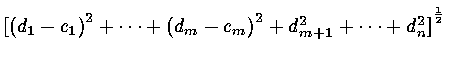where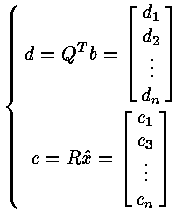The min. of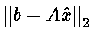is in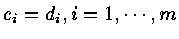and the min. is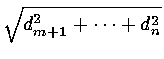( Note : di is fixed , ci is changing .)

Full-Rank Case :

S =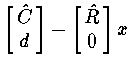=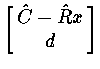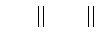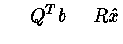Thus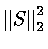=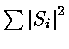=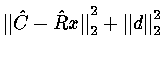( When the min. product in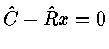, then x is the solve of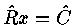P160 3.36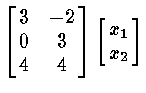=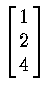C = QT b =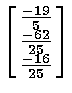R =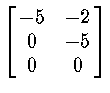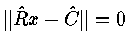Ax = bQT A x = QT b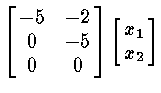=solution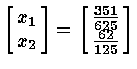Least Square Error :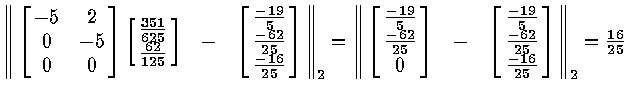Rank-Deficient Case

If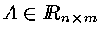, n > m ,rank(A) = r < m , then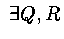s.t.  A = QR ,  where  R =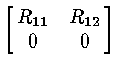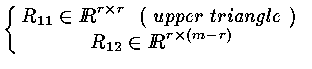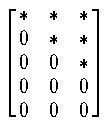rank(3)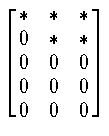rank(A) = 2

QT A x = QT bRx = QT b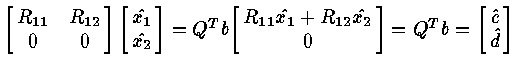Least Square Error :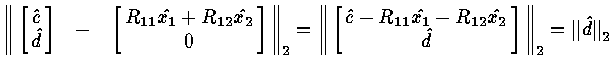( If we choose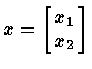s.t.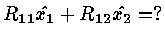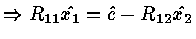)

Rank is small , Least Square is large .